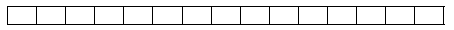Mathematical and Physical Journal
for High Schools
Issued by the MATFUND Foundation
 Already signed up? New to KöMaL?

#Problem K. 475. (November 2015)

K. 475. In the fields in the table (see the figure), enter the integers 1 to 15 so that the sum of the two numbers in any two adjacent field is a perfect square.(6 pont)

Deadline expired on December 10, 2015.

### Statistics:

 159 students sent a solution. 6 points: 95 students. 5 points: 23 students. 4 points: 17 students. 3 points: 17 students. 0 point: 5 students. Unfair, not evaluated: 2 solutionss.

Problems in Mathematics of KöMaL, November 2015